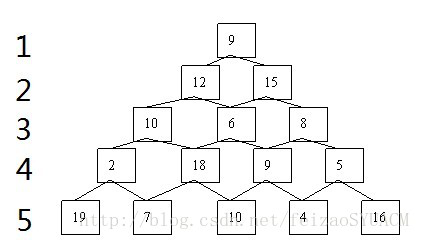# 动态规划算法

1. 定义

2. 概念

3. 求解基本步骤

4. 谈谈动态规划与其他算法之间的联系和区别

5. 动态规划迷思

6. 动态规划解决方法辨析

7. 对动态规划总结性理解

1. 数塔问题

2. DAG

3. 多阶段决策问题

4. LIS

5. LCS

6. LCIS

1.  01背包

2.  完全背包

3.  多重背包

4.  二进制优化

5.  混合背包

6.  多条件分类01背包

7.  01背包+第k优解

「它们加起来是多少哇？」

（数了一会…）「8 ！」

「现在呢？」

（迅速地）「9 ！」

「为什么小猿这么快就知道了呢？」

「因为你刚刚加了 1 啊~」

「所以只要记得之前的结果，就不用再计一次数啦。」

1.  定义

原问题的解如何由子问题的解组合而成。

2.  概念

1）动态规划是运筹学中用于求解决策过程中的最优化数学方法。 当然，我们在这里关注的是作为一种算法设计技术，作为一种使用多阶段决策过程最优的通用方法。

2）如果问题是由交叠的子问题所构成，我们就可以用动态规划技术来解决它，一般来说，这样的子问题出现在对给定问题求解的递推关系中，这个递推关系包含了相同问题的更小子问题的解。动态规划法建议，与其对交叠子问题一次又一次的求解，不如把每个较小子问题只求解一次并把结果记录在表中（动态规划也是空间换时间的），这样就可以从表中得到原始问题的解。

因为动态规划的实现有很成熟的两类方法：

①“自顶向下”（top-down dynamic programming） 的实现方式；

②“自底向上” （bottom-up dynamic programming）的实现方式；

所以，解动态规划问题本质还是找出“原问题的解如何由子问题组合而成”，这是动态规划的本质

①对问题状态的定义；

②对状态转移方程的定义；

3.  求解基本步骤

1）划分阶段：按照问题的时间或空间特征，把问题分为若干个阶段。在划分阶段时，注意划分后的阶段一定要是有序的或者是可排序的，否则问题就无法求解

2）确定状态和状态变量：将问题发展到各个阶段时所处于的各种客观情况用不同的状态表示出来。当然，状态的选择要满足无后效性

3）确定决策并写出状态转移方程：因为决策和状态转移有着天然的联系，状态转移就是根据上一阶段的状态和决策来导出本阶段的状态。所以如果确定了决策，状态转移方程也就可写出。但事实上常常是反过来做，根据相邻两个阶段的状态之间的关系来确定决策方法和状态转移方程。

4）寻找边界条件：给出的状态转移方程是一个递推式，需要一个递推的终止条件边界条件

①分析最优解的性质，并刻画其结构特征；

②递归的定义最优解；

③以自底向上或自顶向下的记忆化方式（备忘录法）计算出最优值；

④根据计算最优值时得到的信息，构造问题的最优解。

4.  谈谈动态规划与其他算法之间的联系和区别（要求较高，初学者可跳过）

LIS(i)=max{LIS(j)+1}       j<i and a[j]<a[i]

5.  动态规划迷思

a. “缓存”，“重叠子问题”，“记忆化”：

上述的需要再次计算的“第99项”，就叫“重叠子问题”。如果没有计算过，就按照递推式计算，如果计算过，直接使用，就像“缓存”一样，这种方法，叫做“记忆化”，这是递推式求解的技巧。这种技巧，通俗的说叫“花费空间来节省时间”。都不是动态规划的本质，不是动态规划的核心。

b. “递归”：

c. “无后效性“，“最优子结构”：

在对状态和状态转移方程的定义过程中，满足“最优子结构”是一个隐含的条件（否则根本定义不出来）。

6.  动态规划解决方法辨析

“自顶向下”的实现方式 和 “自低向上”的实现方式各有什么优缺点，我的理解如下。

“自顶向下”（top-down dynamic programming）：

①能方便的得到递归公式，并用递归函数实现；

②保持了递归实现的代码结构，逻辑上容易理解；

③过程中只计算需要计算的子结果；

“自底向上”（bottom-up dynamic programming）：

①需要设计数据结构来完成自底向上的计算过程。逻辑上相对不那么直观；

7.  对动态规划总结性理解

（1）“动态规划是什么”？

（2）为什么需要使用动态规划？

1.  数塔问题

（1）练手

F(n)=F(n-1) + F(n-2);      其中F(1)=1, F(2)=2

  int  solution(int n)
{
if(n>0 && n<2)
return n;
return solution(n-1) + solution(n-2);
}  

  int dp;
int  solution(int n)
{
if(n>0 && n<2)
return n;
if(dp[n]!=0)
return dp[n];
dp[n] = solution(n-1) + solution(n-2);
return  dp[n];
}  

  int  solution(int n)
{
int dp[n+1];
dp=1;dp=2;
for (i = 3; i <= n; ++i)
dp[n] = dp[n-1] + dp[n-2];
return  dp[n];
}  

（2）实践dp[i][j] = max(dp[i+1][j], dp[i+1][j+1]) + data[i][j])

9

12    15

10    6     8

2     18    9     5

19    7     10    4   16

59

50   49

38   34    29

21   28    19    21

19   7     10    4    16

2.  DAG

DAG也叫有向无环图，是学习动态规划的基础。很多问题都可以转化为DAG上的最长路、最短路或路径计数问题。

3.  多阶段决策问题

d[i][j]为到达第i行第j列的最小（最大）花费

4.  LIS

LIS是最长上升子序列的缩写。给定n个整数A1，A2，…，An，按从左到右的顺序选出尽量多的整数，组成一个上升的子序列（子序列可以理解为：删除0个或者多个数，其他数的顺序不变），例如序列：1,6,2,3,7,5，可以选出上升子序列1,2,3,5，也可以选出1,6,7,但前者更长。注意，选出的上升子序列中相邻元素不能相等。

d[i]为以i结尾的最长上升子序列的长度

d[i]=max(0,d[j]|j<i,Aj<Ai)+1

max(d[i])

5.  LCS

LCS全称叫做最长公共子序列问题。给定两个子序列A和B，求长度最大的公共子序列。

d[i][j]为A1,A2,...,Ai和B1,B2,...Bj的LCS长度

（1）a[i] == b[j]时

d[i][j] = d[i-1][j-1]+1;

（2）a[i] != b[j]时

d[i][j] = max(d[i-1][j],d[i][j-1]);

6.  LCIS

LCS是增加的一个内容，叫做最长递增公共子序列

d[i][j]为以a串的前i项和b串的前j项且以b[j]为结尾构成的LCIS的长度

（1）a[i] == b[j]时

d[i][j] = max+1;

（2）a[i] >  b[j]时

max = max(max,d[i-1][j]);

（1）a[i] == b[j]时

d[j]  max+1;

（2）a[i] >  b[j]时

max = max(max,d[j]+1);

1.  01背包

dp[i][j]表示把前i个物品装入容量为j的背包的最大总价值，则状态转移方程可以表示为：

dp[i][j]=min(dp[i-1][j],dp[i-1][j-w[j]]+v[j])；

dp[j]=min(dp[j],dp[j-w[i]]+v[i])；

2.  完全背包

for(int i=0;i<n;i++)
for(int j=0;j<=V;j++)    //将01背包降一维的逆序改为顺序即可
d[j]=max(d[j],d[j-v[i]]+w[i]);

（想想为什么可以这样做？试着从贪心的角度去分析）

3.  多重背包

 for(int i=0;i<n;i++)
for(int j=1;j<=k[i];j++)      //多重背包，最简单的做法就是插入一个for循环
for(int p=C;p>=v[i];p--)      //多重背包变01背包，降一维要逆序
dp[p]=max(dp[p],dp[p-v[i]]+w[i]); 

4.  二进制优化

1,2,4,...,2^(k-1)，num[i]-2^k+1

k是满足num[i]-2^k+1的最大整数。

void ZeroOne_Pack(int v,int w,int m)
{
for(int i=C; i>=v; i--)
dp[i] = max(dp[i],dp[i-v] + w);
}

void Complete_Pack(int v,int w,int C)
{
for(int i=v; i<=C; i++)
dp[i] = max(dp[i],dp[i-v] + w);
}

memset(dp,0,sizeof(dp));
for(int i=1; i<=n; i++)
{
if(num[i]*c[i] > C)
Complete_Pack(c[i],w[i],C);
else
{
int k = 1;
while(k < num[i])
{
ZeroOne_Pack(k*c[i],k*w[i],C);
num[i] -= k;
k *= 2;
}
ZeroOne_Pack(num[i]*c[i],num[i]*w[i],C);
}
}
return dp[m];

5.  混合背包

2..N+1行：Wi,Ci,Pi，前两个整数分别表示每个物品的重量，价值，第三个整数若为0，则说明此物品可以购买无数件，若为其他数字，则为此物品可购买的最多件数(Pi)。

for(int i=0;i<n;i++)
{
if(num[i]==0)
for(int  j=0;j<V;j++)        //完全背包
d[j]=max(d[j],d[j-v[i]]+w[i]);
else  if(num[i]==1)
for(int j=V;j>=0;j--)       //01背包
d[j]=max(d[j],d[j-v[i]]+w[i]);
else
多重背包（略）；
}

6.  多条件分类01背包

s=0说明这m件事中最少得做一件;
s=1说明这m件事中最多只能做一件;
s=2说明这m件事你可以做也可以不做;

dp[i][j] 表示处理完前面i个工作集所花时间不超过j的最大快乐值

①当s==0 的时候，表示这个集合内的m件事情至少做一件，也就分两种情况比较：

1）这个集合中m件事情已经选择其中k件做了（k>=1）;

dp[i][j]=max(dp[i][j],dp[i][j-cost]+val);

2）这个集合中m件事情还没有做过一件；

dp[i][j]=max(dp[i][j],dp[i-1][j-cost]+val);

②当s==1的时候，表示这个集合内的m件事情最多做一件，如果现在还有挑选的余地，就已经说明这个集合中的m件事情还没有做过一件;

dp[i][j]=max(d[i][j],dp[i-1][j-cost]+val);

③当s==2的时候，表示这个集合内的m件事情可以不做任何一件，也可以做任何多件；

dp[i][j]=max(dp[i][j],dp[i][j-cost]+val);

memset(dp,0,sizeof(dp));
for(int i=1;i<=n;i++)
{
cin>>m>>s;
for(int j=0;j<=t;j++)
dp[i][j]=s==0?-INF:dp[i-1][j]; //如果s==0 则至少需要选择一个的01背包 若剩下的时间已经不够了 那么就需要输出-1 因此初始化-INF
for(int k=0;k<m;k++)
{
cin>>cost>>val;
for(int j=t;j>=cost;j--)         //都选择逆序枚举
{
if(s==0)          //至少选择一个的01背包
dp[i][j]=max(max(dp[i][j],dp[i][j-cost]+val),dp[i-1][j-cost]+val);
else if(s==1)     //最多选择一个的01背包
dp[i][j]=max(dp[i][j],dp[i-1][j-cost]+val);
else               //选择任意多个的01背包
dp[i][j]=max(dp[i][j],dp[i][j-cost]+val);
}
}
}
int ans=max(dp[n][t],-1);

7.  01背包+第k优解

也就是说，相当于全年级有两个班，要求年级排名第k，那么只要知道这两个班的班排名前k就行了。

memset(dp,0,sizeof(dp));
for(int i=0;i<n;i++)
{
for(int j=v;j>=vol[i];j--)
{
for(kk=1;kk<=k;kk++)
{
A[kk]=dp[j-vol[i]][kk]+val[i];   //A[] 记录选择第i个物品的从大到小的排列
B[kk]=dp[j][kk];                 //B[] 记录不选择第i个物品的从大到小的排列
}
A[kk]=B[kk]=-1;
a=b=c=1;
while(c<=k&&(A[a]!=-1||B[b]!=-1))
{
dp[j][c]=A[a]>B[b]?A[a++]:B[b++];        //d[i][j] 记录体积为 i 的时候的第 j 优解
if(dp[j][c]!=dp[j][c-1])          //去重操作
c++;
}
}
}

04-2515万+02-175296
02-275万+
10-13109
11-051095
08-093万+
10-1120万+
07-111万+
05-025230
10-272万+
06-111894
10-251万+
02-295万+
11-16178
07-191万+
06-059958
07-28832
©️2020 CSDN 皮肤主题: 精致技术 设计师:CSDN官方博客